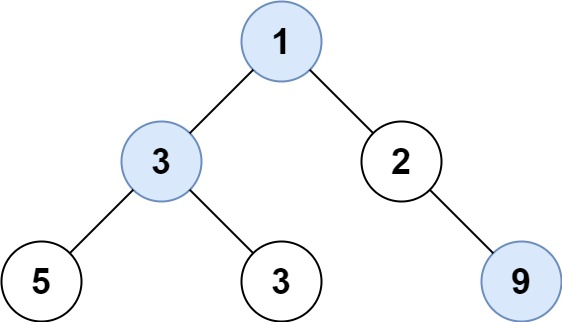## C# || How To Find Largest Value In Each Binary Tree Row Using C#The following is a module with functions which demonstrates how to find the largest value in each binary tree row using C#.

1. Largest Values – Problem Statement

Given the root of a binary tree, return an array of the largest value in each row of the tree (0-indexed).

Example 1:``` Input: root = [1,3,2,5,3,null,9] Output: [1,3,9] ```

Example 2:

``` Input: root = [1,2,3] Output: [1,3] ```

2. Largest Values – Solution

The following is a solution which demonstrates how to find the largest value in each binary tree row.

``` 2. Largest Values - Solution C# // ============================================================================ // Author: Kenneth Perkins // Date: Oct 28, 2023 // Taken From: http://programmingnotes.org/ // File: Solution.cs // Description: Demonstrates how to find the largest value binary tree row // ============================================================================ /** * Definition for a binary tree node. * public class TreeNode { * public int val; * public TreeNode left; * public TreeNode right; * public TreeNode(int val=0, TreeNode left=null, TreeNode right=null) { * this.val = val; * this.left = left; * this.right = right; * } * } */ public class Solution { public IList<int> LargestValues(TreeNode root) { if (root == null) { return new List<int>(); } var ans = new List<int>(); var queue = new Queue<TreeNode>(); queue.Enqueue(root); while (queue.Count > 0) { int currMax = int.MinValue; for (int i = queue.Count -1; i >= 0; --i) { var node = queue.Dequeue(); currMax = Math.Max(currMax, node.val); if (node.left != null) { queue.Enqueue(node.left); } if (node.right != null) { queue.Enqueue(node.right); } } ans.Add(currMax); } return ans; } }// http://programmingnotes.org/ 123456789101112131415161718192021222324252627282930313233343536373839404142434445464748 // ============================================================================//    Author: Kenneth Perkins//    Date:   Oct 28, 2023//    Taken From: http://programmingnotes.org///    File:  Solution.cs//    Description: Demonstrates how to find the largest value binary tree row// ============================================================================/** * Definition for a binary tree node. * public class TreeNode { *     public int val; *     public TreeNode left; *     public TreeNode right; *     public TreeNode(int val=0, TreeNode left=null, TreeNode right=null) { *         this.val = val; *         this.left = left; *         this.right = right; *     } * } */public class Solution {    public IList<int> LargestValues(TreeNode root) {        if (root == null) {            return new List<int>();        }         var ans = new List<int>();        var queue = new Queue<TreeNode>();        queue.Enqueue(root);         while (queue.Count > 0) {            int currMax = int.MinValue;            for (int i = queue.Count -1; i >= 0; --i) {                var node = queue.Dequeue();                currMax = Math.Max(currMax, node.val);                 if (node.left != null) {                    queue.Enqueue(node.left);                }                if (node.right != null) {                    queue.Enqueue(node.right);                }            }            ans.Add(currMax);        }        return ans;    }}// http://programmingnotes.org/ ```

QUICK NOTES:
The highlighted lines are sections of interest to look out for.

The code is heavily commented, so no further insight is necessary. If you have any questions, feel free to leave a comment below.

Once compiled, you should get this as your output for the example cases:

``` [1,3,9] [1,3] ```